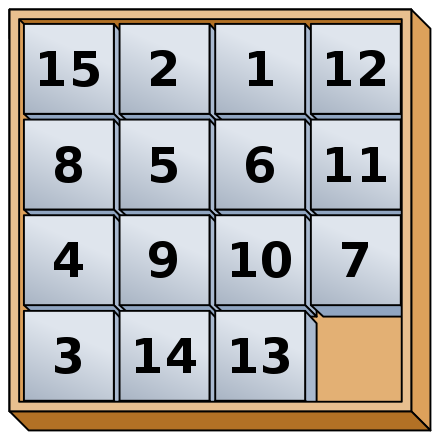CS 440/ECE 448
Margaret Fleck

## What makes a heuristic "reasonable"?

Heuristic functions for A* need to have two properties

• quick to compute
• underestimates of the true distance to the goal.

In the context of $$A^*$$ search, being an underestimate is also known as being "admissible."

Heuristics are usually created by simplifying ("relaxing") the task, i.e. removing some constraints. For search in mazes/maps, we can use straight line distance, so ignoring

• obstacles (e.g. in a maze), and
• the fact that roads may be missing or not straight or have different speed limits (e.g. in a graph-like road map).

All other things being equal, an admissible heuristic will work better if it is closer to the true cost, i.e. larger. A heuristic function h is said to "dominate" a heuristic function with lower values.

For example, suppose that we're searching a digitized maze and the robot can only move in left-right or up-down (not diagonally). Then Manhattan distance is a better heuristic than straight line distance. The Manhattan distance between two points is the difference in their x coordinates plus the difference in their y coordinates. If the robot is allowed to move diagonally, we can't use Manhattan distance because it can overestimate the distance to the goal.

Another classical example for $$A^*$$ is the 15-puzzle. A smaller variant of this is the 8-puzzle..Two simple heuristics for the 15-puzzle are

• Number of misplaced tiles
• Sum of the Manhattan distances between each tile's current location and goal location.

See 8/15-puzzle demo by Julien Dramaix

Search stops when goal state becomes top option in frontier (not when goal is first discovered). Suppose we have found goal with path P and cost C. Consider a partial path X in the frontier. Its estimated cost must be $$\ge C$$. Since our heuristic is admissible, the true cost of extending X to reach the goal must also be $$\ge C$$. So extending X can't give us a better path than P.
$$A^*$$ decays gracefully if admissibility is relaxed. If the heuristic only slightly overestimates the distance, then the return path should be close to optimal. This is called "suboptimal" search. Non-admissible heuristics are particularly useful when there are many alternate paths of similar (or even the same) cost, as in a very open maze. The heuristic is adjusted slightly to create a small preference for certain of these similar paths, so that search focuses on extending the preferred paths all the way out to the goal. See Amit Patel's notes for details.